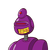# a dining table in the shape of a rectangle ABCD has sides of length 72cm and ×cm, and the length of the diagonal BD is 78cm . find

a dining table in the shape of a rectangle ABCD has sides of length 72cm and ×cm, and the length of the diagonal BD is 78cm . find the value of ×.​

### 1 thought on “a dining table in the shape of a rectangle ABCD has sides of length 72cm and ×cm, and the length of the diagonal BD is 78cm . find”

1.we know that

(2x+12)^2 + x^2 = (2x+18)^2

expand all that and solve the quadratic, and you get

x = 30

check: 30^2 + 72^2 = 78^2

That’s just 6 times a 5-12-13 triangle